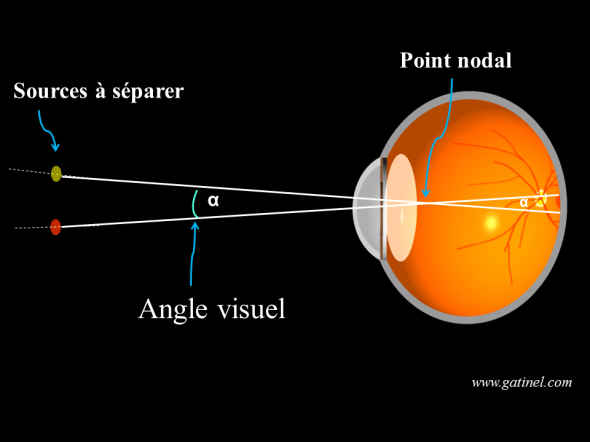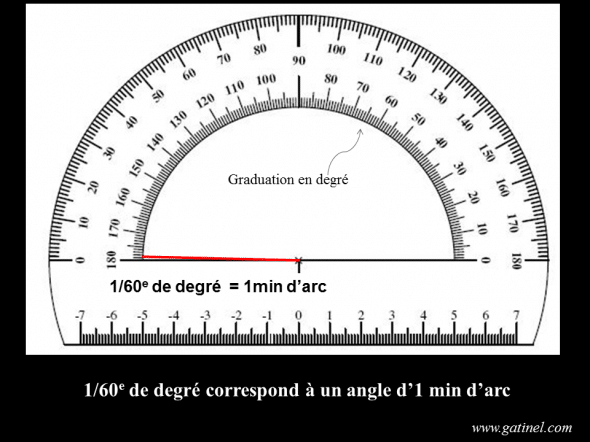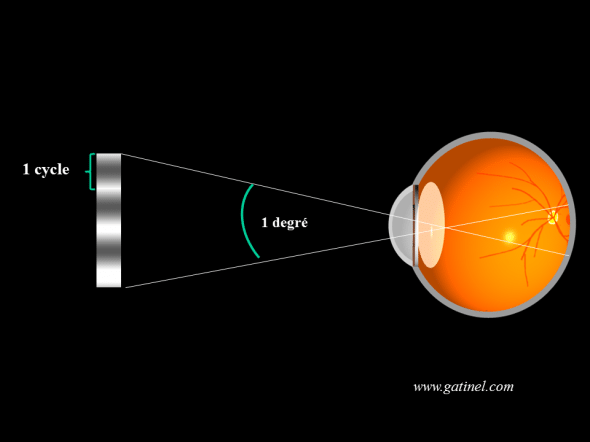+ +

# Visual angle

The notion of Visual angle or apparent angle comes up frequently in physiological optics, including to characterize the resolving power of the eye (Visual acuity). The apparent Visual angle and angular size is used to free itself from the distance between the eye and the observed target: a diameter of 1 cm at a distance of 1 meter will be imaged on the retina with a same size of a piece of a diameter of 2 cm at a distance of 2 metres.

### Apparent angle

The apparent angle integrates in a same size distance and size of the object. It can be used to quantify the power of eye resolution, which corresponds to the minimum angle below which we cannot separate two distinct point light sources (example: a pair of double stars). The term is also used of "resolving power.The Visual angle is formed by the two sources and the nodal point, occupying the Summit of this angle. The nodal point is located about 17 mm from the surface of the retina. The minimum angle to resolve as separate two frequent sources corresponds to the resolving power of the eye.

### Visual angle: units

The apparent Visual angle is expressed in different units: radians (for calculations), ('big' angles) degrees, or minutes and seconds of arc ('small ' angles).  Fortunately, there are conversion formulas that allow to move from one unit to the other.

By following the path of a 'unit' (of RADIUS 1) circle, a 360 ° rotation is needed to achieve a full turn. The perimeter of this circle worth 2 Pi, we easily get the value in radians of an angle expressed in degrees: A (rad) = Pix (deg) / 180.

A radian value matches the angle which is formed by an arc of the same length as the RADIUS that generates it.  When an object is part of an angle small (ex: 1 °, area of study of the OPD, or PI/180 = 0.017 radians), one can calculate the actual size of an object which we know the distance to the eye by multiplying it by the angle in radians (figure).

A degree is divided into 60 minutes of arc; each arc minute is divided into 60 seconds of arc.A degree of angle can be subdivided into 60 minutes of arc, which can each be divided into 60 seconds of arc.

The measurement of Visual acuity is based partly on the resolving power of the eye, which allows him to see distinct two points separated by a Visual angle which cannot be reduced under sentence to "confuse" the images (which are more separated).

Visual acuity is defined as the inverse of the value of the minimum angle of resolution expressed in minutes of arc, often referred to by the acronym MAR (Minimum Resolution Angle).

A resolution of 1 minute of arc power corresponds to be able to see two points as "distinct" that under tend an angle of 1/60e degree. It is a theoretical acuity of 1/1 = 10/10. A resolving power of 1.2 minutes of arc is a Visual acuity of 1 / 1.25 = 8/10, and 2 minutes of arc to a Visual acuity of 1/2 = 5/10.

Logically, more market increases, more Visual acuity decreases, and vice versa.

The visual angle of the lunar disk is half a degree, approximately 30 minutes of arc. This is approximately the angle under which the nail of an index saw elongated arm. The apparent diameter of Jupiter seen from Earth is close to 30 seconds of Arc, the SCNV resolution limit of the human eye; To the naked eye, Jupiter actually appears as a record and not a point for an observer with a good visual acuity.

### Visual angle and cycles

The splitter power of the eye can also be expressed as the amount of "details" that it is able to "solve" when they are enrolled in a given angle (eg: 1 °). For example, an elemental detail consists of a "cycle", which connects two different luminance ranges (see also: spatial frequencies). The smaller the cycle, the more you can repeat it in a degree of angle (the cycles are all the more "fine" they are many). The resolution capacity of an eye can be quantified by the number of cycles it is able to discern in an apparent degree of visual angle. The contrast within each cycle, i.e. the difference between the minimum and maximum luminances, obviously plays an important role in this capacity. A visual acuity of 10/10 is equivalent to the power to discern 30 cycles (maximum contrast) per degree. A sharpness of 20/10 allows to solve 60 cycles per degree.In this example (drawing not to scale), ago 3 cycles per degree. If Visual acuity was of 3 cycles per degree (i.e. about 1/10th), this would mean that the eye cannot resolve a pattern more thin, consisting more of 3 cycles per degree.

### 5 Responses to "visual Angle"

1.Jean-Luc Mainardi says:

Good morning, sir
I'm an Amateur astronomer.
Soon, May 9th the planet Mercury will pass in front of the sun;
The size of the planet's disk is 12 minutes of arc so well above the limit of perception of the eye.
But we won't be able to see her with the naked eye!
= = > In the world of astronomy we recommend a magnification of at least 40 x for
Sighting (an apparent angle of 40x 12 ' = 480 ' = 8 °!!!
How to explain such a difference in the abilities of the eye especially as the eye works with a contrast close to 1 in this case.
Jean-Luc Mainardi

2.Jean-Luc Mainardi says:

Hello Sir,

I realize after the fact that I made a profound mistake: I confused the minutes of arc with the arc seconds!
Mercury, during its passage in front of the sun will have an angular diameter of 12 seconds of Arc, which, of course, is very much below the detection power of the eye.
The use of a magnifying astronomical instrument at least 40x is therefore recommended to see this tiny black point on the surface of the sun.
I beg you to consider my previous question as null and void.

We, amateur astronomers, always refer to the works of Albert Arnulf
And the conclusions he draws from it with regard to the vision with the naked eye in the instruments (works of 1937!)
Very cordially
Jean-Luc Mainardi

3.Dr. Damien Gatinel says:

Thanks for your comments lit up, I am fan of astronomy (amateur) and am delighted to be able to observe, probably by interposed journals, the images of this partial 'eclipse' Mercurian. Should be effectively to the human eye acuity superior to discern the edges of the disk of mercury three times. That said, acuity of detection, thinner (10 seconds of arc), would allow in theory to collect mercury "a point" in front of the Sun.

4.Adeline says:

Hello
And thank you for your site very well done.
It seems to me that there is a small shell in the last sentence "A acuity of 20/10 allows to solve 20 cycles per degree." (It's 60 CPD, not 20, isn't it?).
Sincerely,
5.Dr. Damien Gatinel says: